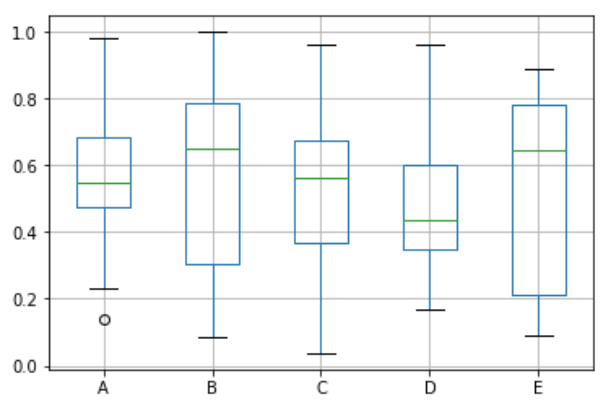# (26)Python箱形图

## 绘制箱线图

Boxplot可以绘制调用`Series.box.plot()``DataFrame.box.plot()``DataFrame.boxplot()`以可视化每列中值的分布。

``````import pandas as pd
import numpy as np
df = pd.DataFrame(np.random.rand(10, 5), columns=['A', 'B', 'C', 'D', 'E'])
df.plot.box(grid='True')
```Python```